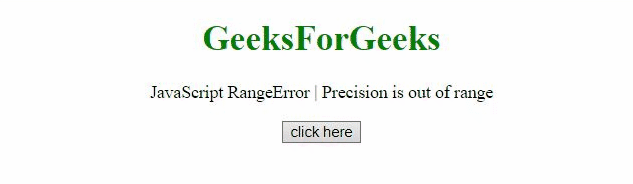# JavaScript RangeError | Precision is out of range

• Last Updated : 22 Jul, 2020

This JavaScript exception precision is out of range occurs if a number which is outside the range of 0 and 20 (or 21) is passed into toFixed(), toPrecision(), or toExponential() method.

Error Message:

RangeError: The number of fractional digits is out of range (Edge)
RangeError: The precision is out of range (Edge)
RangeError: precision {0} out of range (Firefox)
RangeError: toExponential() argument must be between 0 and 20 (Chrome)
RangeError: toFixed() digits argument must be between 0 and 20 (Chrome)
RangeError: toPrecision() argument must be between 1 and 21 (Chrome)

Error Type:

`RangeError`

What Happened?

There is a precision argument that is out of range in one of the methods, toExponential(), toFixed(), and toPrecision().

Example 1: In this example, the RangeError occurred as -100 to toFixed() is passed.

## HTML

 ```<``html``>`` ` `<``head``>``    ``<``script` `src``=``"https://code.jquery.com/jquery-3.5.0.js"``>``    `````` ` `<``body` `style``=``"text-align:center;"``>``    ``<``h1` `style``=``"color:green;"``>``        ``GeeksForGeeks``    ```` ` `    ``<``p``>``        ``JavaScript RangeError | Precision is out of range``    ```` ` `    ``<``button` `onclick``=``"Geeks();"``>``        ``click here``    ```` ` `    ``<``p` `id``=``"GFG_DOWN"``>`` ` `    ``<``script``>``        ``var el_down = document.getElementById("GFG_DOWN");``        ``function Geeks() {``            ``try {``                ``3.54.toFixed(-100);``                ``el_down.innerHTML = "'Precision out of range'"``                        ``+ " error has not occurred";``            ``} catch (e) {``                ``el_down.innerHTML = "'Precision out of range'"``                        ``+ " error has occurred";``            ``}``        ``} ``    `````` ` ``

Output:Example 2: In this example, the argument passed to toExponential() is -4, So the RangeError has occurred.

## HTML

 ```<``html``>`` ` `<``head``>``    ``<``script` `src``=``"https://code.jquery.com/jquery-3.5.0.js"``>``    `````` ` `<``body` `style``=``"text-align:center;"``>``    ``<``h1` `style``=``"color:green;"``>``        ``GeeksForGeeks``    ```` ` `    ``<``p``>``        ``JavaScript RangeError | ``        ``Precision is out of range``    ```` ` `    ``<``button` `onclick``=``"Geeks();"``>``        ``click here``    ````    ``<``p` `id``=``"GFG_DOWN"``>``    ```` ` `    ``<``script``>``        ``var el_down = document.getElementById("GFG_DOWN");``        ``function Geeks() {``            ``try {``                ``77.1234.toExponential(-4);``                ``el_down.innerHTML = "'Precision out of range'"``                        ``+ " error has not occurred";``            ``} catch (e) {``                ``el_down.innerHTML = "'Precision out of range'"``                        ``+ " error has occurred";``            ``}``        ``} ``    `````` ` ``

Output:Example 3: In this example, the RangeError occurred as -1 to toPrecision() is passed.

## HTML

 ```<``html``>`` ` `<``head``>``    ``<``script` `src``=``"https://code.jquery.com/jquery-3.5.0.js"``>``    `````` ` `<``body` `style``=``"text-align:center;"``>``    ``<``h1` `style``=``"color:green;"``>``        ``GeeksForGeeks``    ```` ` `    ``<``p``>``        ``JavaScript RangeError | ``        ``Precision is out of range``    ```` ` `    ``<``button` `onclick``=``"Geeks();"``>``        ``click here``    ````    ``<``p` `id``=``"GFG_DOWN"``>``    ```` ` `    ``<``script``>``        ``var el_down = document.getElementById("GFG_DOWN");``        ``function Geeks() {``            ``try {``                ``5643.9.toPrecision(-1);``                ``el_down.innerHTML = "'Precision out of range'"``                        ``+ " error has not occurred";``            ``} catch (e) {``                ``el_down.innerHTML = "'Precision out of range'"``                        ``+ " error has occurred";``            ``}``        ``} ``    `````` ` ``

Output:My Personal Notes arrow_drop_up